# 16.3: Oxidation States - Electron Bookkeeping

Redox reactions are all about electrons being transferred from one substance to another, so it would be useful if we had a system for keeping track of what gains and what loses electrons, and how many electrons are involved. We do - our record-keeping system is called Oxidation Numbers. You may remember from earlier chemistry classes something called electronegativity.

Electronegativity

The ability of a bonded atom to attract shared electrons towards itself

When two atoms of different elements are bonded together by a covalent bond (sharing electrons), the electrons are generally not shared equally between the two atoms due to differences in their electronegativity. Think of this as a tug-of-war for electrons. Sometimes both atoms pull with equal strength on shared electrons; other times there is a clearly stronger player who will pull the electrons closer to itself.

Consider the bond between a hydrogen atom (with one valence electron) and an oxygen atom (with its six valence electrons):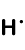+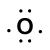=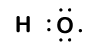Because oxygen has a higher electronegativity than hydrogen, the shared electrons are closer to the oxygen atom than to the hydrogen atom. This is not the total transfer of electrons that would create an ion, but partial charges do form - the hydrogen end of the bond is partially positive (+1) because it has partially lost one electron, and the oxygen end of the H–O is partially negative (-1) because it has partially gained the one electron from hydrogen: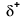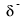Our molecule is incomplete however, because there is a lone electron around oxygen. Let's add one more hydrogen to complete our water molecule: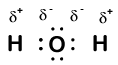We see that each of the two hydrogen has "lost" one electron to oxygen. Oxygen has "gained" two electrons - one from each hydrogen. Again, these are not true ions but it is useful to think of them in the same way.

Charges given to atoms in a molecule in this way are called oxidation numbers. We can use oxidation numbers to keep track of where electrons are in a molecule, and how they move during a reaction. In our water example, hydrogen is assigned an oxidation number of +1 because each individual hydrogen has "lost" one electron. Oxygen has an oxidation number of +2 because the single oxygen atom has "gained" a total of two electrons, one from each hydrogen.

Here is another molecule involving hydrogen and oxygen - hydrogen peroxide, H2O2: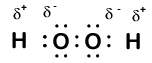In hydrogen peroxide, each hydrogen still has an oxidation number of +1 because each hydrogen "gives up" a single electron to oxygen. Oxygen, however, now has an oxidation number of -1 because each oxygen gains just one electron from its neighboring hydrogen. The electrons between the two identical oxygen atoms are shared equally, so there is no partial charge resulting from that bond.

Oxidation Numbers

A positive or negative number assigned to an atom in a molecule or ion that reflects a partial gain or loss of electrons

Knowing the oxidation number of each individual element in a molecule will be a key step in our understanding of redox reactions. Fortunately it will not usually involve drawing electron dot diagrams. Instead there are a series of rules that we can use to determine oxidation numbers. Here are the main rules:

1. The oxidation number of a pure element (by itself, and not an ion) is zero.
 Element Oxidation Number Na 0 H2 0 O2 0 P4 0
2.

The oxidation number of a monatomic ion (by itself or as part of an ionic compound) is equal to its charge.

Alkali metals - elements in the first column of the periodic table - will always have an oxidation number of +1; Alkali metals (column 2) are almost always +2

 Ionic Compound Ions Charge Oxidation Number NaCl Na+ +1 +1 Cl- -1 -1 Mg3N2 Mg+2 +2 +2 N-3 -3 -3
3. The oxidation number of hydrogen is almost always +1 when it is in a compound.
 Compound element Oxidation Number HCl H +1 Cl -1 H2S H +1 S -2
4.

The oxidation number of oxygen is almost always -2 when it is in a compound

The exceptions:

• peroxides, such as hydrogen peroxide. In peroxides oxygen has an oxidation number of -1.
• when oxygen is combined with fluorine it's oxidation number is +2
 Compound element Oxidation Number MgO magnesium oxide Mg +2 O -2 Na2O sodium oxide Na +1 O -2 Na2O2 sodium peroxide Na +1 O -1
5.

The sum of the oxidation numbers in a compound is zero.

To determine the oxidation number of Mn in Mn2O7 we must work backwards:

• We know each oxygen is -2 (Rule 4)
• 7 oxygen gives a total of:

-2 × 7 atoms = -14 total

Since the sum of oxidation numbers must be zero, the total oxidation number of Mn must be +14 to cancel out oxygen's -14, but since there are 2 Mn atoms, each individual atom will have an oxidation number of +7:

 +14 total 2 Mn atoms = +7
 Compound element Oxidation Number Number atoms Total Mg3N2 Mg +2 3 +6 N -3 2 -6 SUM 0 Mn2O7 Mn +7 2 +14 O -2 7 -14 SUM 0 Cl2O3 Cl +3 2 +6 O -2 3 -6 SUM 0

6.

The sum of the oxidation numbers in a polyatomic ion is equal to the charge on that ion.

Again, work backwards to determine the oxidation number of any non-oxygen or non-hydrogen atom.

To determine the oxidation number of Cr in Cr2O72- :

• Oxygen will be -2 (Rule 4), for a total of:

-2 × 7 = -14

• Since the sum of the oxidation numbers will be -2 (the charge on the entire ion), the total for all Cr must be +12 because:

+12 + (-14) = -2

• Since there is are two Cr, each Cr will have an oxidation number of +6
= +7
 +12 2 = +6
 Compound element Oxidation Number Number atoms Total NO3- N +5 1 +5 O -2 3 -6 SUM -1 Cr2O72- Cr +6 2 +12 O -2 7 -14 SUM -2 SO42- S +6 1 +6 O -2 4 -8 SUM -2

It is important to note that oxidation number always refers to each individual atom in the compound, not to the total for that element.

For example in H2O - the total positive "charge" for both hydrogen atoms will be +2 (which balances with the -2 from oxygen), but EACH HYDROGEN has an oxidation number of +1.

## Contributors

• CK-12 Foundation by Sharon Bewick, Richard Parsons, Therese Forsythe, Shonna Robinson, and Jean Dupon.